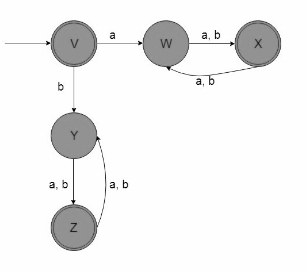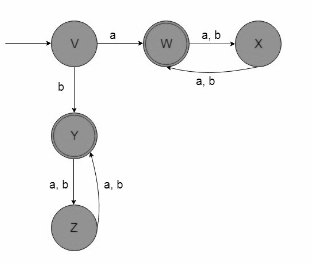# Construct a Finite Automata for the regular expression ((a+b)(a+b))*.

The language for the given regular expression (RE) is as follows −

L={ ε,aa,ab,ba,aaaa,………}## Example

Let the regular expression be ((a+b)(a+b))*(a+b).

Construct the Finite automata for the given regular expression.

First, generate the language for the given Regular Expression −

L={a,d,aaa,bbb,abb,bab,bba,………..}

This is the language of odd length strings

The Finite Automata is as follows −